# JavaScript array contains: How to use array includes() Function

To check if the JavaScript contains any element in the array, use the array.includes() method.

## Javascript array includes

The array includes() is a built-in JavaScript method that defines whether the array contains the specified element or not. The includes() function accepts element and start parameters and returns true or false as output depending on the result.

The includes() method is case sensitive. This post shows that the array contains an object, a string, and a substring.

### Syntax

The syntax of Javascript Array includes() is the following.

`array.includes(element, start)`

### Parameters

The element parameter is required, and it is the element that we need to search.

The start parameter is optional, and the default is 0. It is the position in the array to start the search.

### Return Value

The includes() function returns boolean value: true or false.

### Example

Let’s take an example of Javascript Array Includes.

```// app.js

let arr = ['Gavin', 'Richard', 'Erlich', 'Gilfoyle'];

console.log(arr.includes('Erlich'));```

As we know, Erlich is in the arr. So it will return true.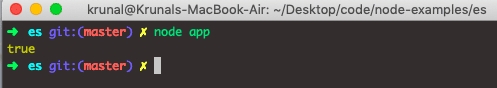If we search for an element that does not exist in an array, it will return the false.

```// app.js

let arr = ['Gavin', 'Richard', 'Erlich', 'Gilfoyle'];

console.log(arr.includes('Jared'));```

See the following output.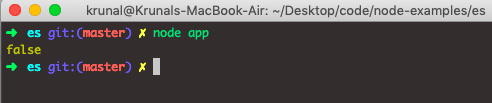When comparing strings and characters, the includes() function is case-sensitive.

Javascript array includes() function uses the sameValueZero algorithm to determine whether the given element is found.

Let’s see other examples.

```// app.js

console.log([1, 2, 3].includes(3, 2));
console.log([19, 21, 46].includes(19, 1));
console.log([19, 21, 46].includes(46, -1));
console.log([1, 2, NaN].includes(NaN));```

The output of the above code is the following.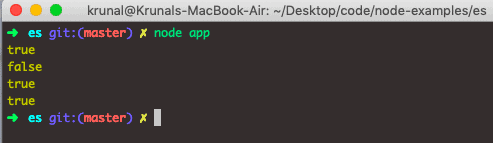If the start position is greater than or equal to the array’s length, false is returned. Therefore, the array will not be searched.

If the start position is negative, the computed index is calculated as a position in an array to search for the element. If the computed index is less or equal to -1 * array .length, an entire array will be searched.

## Javascript array contains

To check if an array contains a value in JavaScript, use the array includes() method. The array includes() method checks whether an array has the item or specified an element or not.

To determine if an array consists of an object in JavaScript, use the array.includes() method. All modern browsers have array includes(), which does precisely that and is widely supported by everyone except IE.

### Syntax of prototype

`Array.prototype.name = value`

Let’s extend the Javascript array prototype.

## Javascript array contains prototype

The prototype constructor allows us to add new properties and methods to the array() object. When constructing the property, ALL arrays will be given the property, and its value, as default. When creating the method, ALL arrays will have this method available.

Note Array.prototype refers not to the single array but to the Array() object itself.

Note: Prototype is the global object constructor available for all JavaScript objects.

See the following code.

```Array.prototype.contains = function (obj) {
var i = this.length;
while (i--) {
if (this[i] === obj) {
return true;
}
}
return false;
}```

Okay, now let’s see an example based on the array.contains() prototype.

```// app.js

Array.prototype.contains = function (obj) {
var i = this.length;
while (i--) {
if (this[i] === obj) {
return true;
}
}
return false;
}

console.log([1, 2, 3].contains(2))
console.log([1, 2, 3].contains(3))
console.log([1, 2, 3].contains(11))```

#### Output

```node app
true
true
false```

The output shows that Javascript Array.contains() prototype that works precisely as expected.

That means we can add this prototype to any file, and we can use the contains() function like it is a native Javascript function.

#### Word of caution

According to some devs, extending the JavaScript Array object is a terrible idea because you introduce new properties (your custom methods) into for-in loops, breaking the existing scripts. A few years ago, the authors of the Prototype library had to re-iterate their library implementation to remove just this kind of thing.

I would recommend a safer free-standing function solution. But, again, the choice is yours as a developer.

### Difference between Array.includes() and Array.indexOf()

The Array.includes() method was introduced in ECMAScript 6. The includes() method is used to find out whether the element is included in the array or not. It returns the boolean value: true or false.

We use array .indexOf() to find out whether the element is present in the array or not. But it doesn’t return a boolean. Instead, it returns the first index of the element found in the array or will return -1 (which represents that the element is not found).

Unlike array indexOf(), which uses Strict Equality Comparison, it includes comparisons using the SameValueZero equality algorithm. That means that you can detect if the array includes a NaN.

## Checking if an array includes an object.

Here, we can use the JS Array filter() method. See the following example.

```// app.js
let list = [{name: 'Krunal'}, {name: 'Ankit'}];

let op = list.filter(data => (data.name == 'Krunal'));

console.log(op)
```

See the output.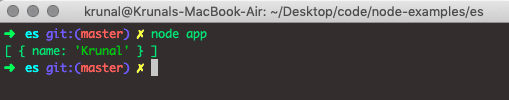We can also use the instanceOf operator to check the type of element. Combining array filter and instanceOf operator, we can check whether the array contains an object or the array includes the object.

See the following code example.

```// app.js
let obj = { author: 'KDL' }
let list = [obj, obj];

let op = list.filter(data => data instanceof Object);
console.log(op)
```

See the following output.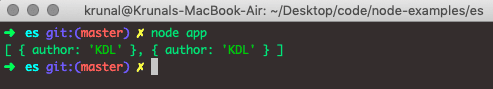So, we can say the array contains an object; otherwise, the filter() method filters out the elements and does not include the final output.

## Checking if an array contains a string.

If we want to find whether an array contains a string, we can use the array indexOf method in Javascript. See the following programming example.

```// app.js
let arr = ['Krunal', 'Ankit', 'Rushabh'];
let arrContainString = (arr.indexOf('Krunal') > -1);
console.log(arrContainString);
```

Now, see the output.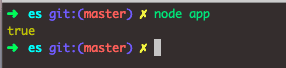That means a specific string is included in the Javascript array.

We can also verify using the instanceOf operator.

## Checking if an array contains a string constructor.

See the following code.

```// app.js

let strA = new String('Krunal');
let strB = new String('Ankit');
let strC = new String('Rushabh');

let arr = [strA, strB, strC];

let arrContainString = arr.filter(element => element instanceof String);
console.log(arrContainString);
```

See the output.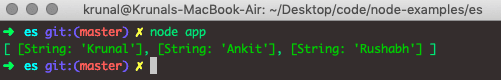## Checking if an array contains the substring

In javascript, you can search an array for a substring match.

```// app.js

let arr = ['Krunal', 'Ankit', 'Rushabh']
let index, value, result;
for (index = 0; index < arr.length; ++index) {
value = arr[index];
if (value.substring(0, 3) === "Ank") {
result = value;
break;
}
}
if (result) {
console.log(result)
}
else {
}

```

See the output.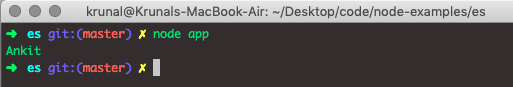In the above code, we have used the for loop and String substring method to check the substring in the array.

## Determine if the Javascript array contains another array.

If we want to determine whether one array contains another in Javascript, we need to use the combination of the array every() and array indexOf() methods. See the code.

```// app.js

let arr = [19, 21, 29, 46];
let arrB = [2, 6, 18, 19, 29, 46, 53, 21];

let op = arr.every(element => arrB.indexOf(element) > -1);
console.log(op);
```

See the output.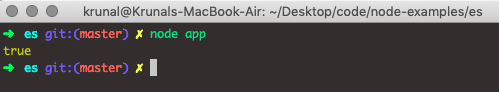In the above code, the second array arrB contains all the elements of the arr; that is why every function returns true.

If one element is missing from arrB, it will output false. So keep in mind that the above way only works if you need to check the whole array and not some array items.

## Javascript array includes multiple conditions.

If we have multiple conditions for JavaScript .includes() method, we can create a custom function that checks if one and only one of the conditions is true. See the following code.

```// app.js

let str = "Friends Don't Lie";
let arr = ['Friends','Eleven', 'Millie']

const contains = (target, pattern) => {
let value = 0;
pattern.forEach(function(word){
value = value + target.includes(word);
});
return (value === 1)
}

console.log(contains(str, arr));
```

So, we have one string and one array in the above example.

Now, we take an array element and check inside the string using the includes() method, and if any element is found in the string, it returns true. We are checking each element using an array.forEach() function.

In our example, Friends is there in the string to return true.

See the output.

```➜  es git:(master) ✗ node app
true
➜  es git:(master) ✗
```

## Determine if the Javascript array contains an object.

To determine if a Javascript array contains an attribute that equals a given value? Well, we have two solutions to define whether an array contains an object with an attribute or not. Therefore, we will not be using Javascript forEach() method.

1. Determine an array containing object using Array.some() method.
2. Determine an array containing object using Array.filter() method.

### Define an array contains object using Array.some() method

Javascript array some() is an inbuilt method that tests whether at least one element in the array passes the test implemented by the provided function. It returns a Boolean value.

See the following code.

```// app.js

info = [
{
name: 'Scarlett',
movie: 'Black Widow'
},
{
name: 'Brie',
movie: 'Room'
},
{
name: 'Angelina',
movie: 'Eternals'
}
]

if (info.some(e => e.name === 'Brie')) {
console.log('Brie won Oscard for the Movie Room')
}```

#### Output

```node app
Brie won Oscard for the Movie Room```

This is the most exact answer because, i.e., “check if something exists”, implying a bool result. This will be true if there are any names with ‘Brie’ value objects, false otherwise.

### Define an array contains object using Array.filter() method

Javascript array filter() is a built-in method that creates an array filled with elements that pass a test (provided as a function).

See the following code.

```// app.js

info = [
{
name: 'Scarlett',
movie: 'Black Widow'
},
{
name: 'Brie',
movie: 'Room'
},
{
name: 'Angelina',
movie: 'Eternals'
}
]

if (info.filter(e => e.name === 'Brie').length > 0) {
console.log('Brie won Oscard for the Movie Room')
}```

#### Output

```node app
Brie won Oscard for the Movie Room```

The js filter() function returns an array of all ‘Brie’ objects, even if only one (will return a one-element array). But we have just tested if it is true or not.

It will execute the if condition based on the true or false, which is all we want. Next, we want to test if the array contains a specific object or not.

That is it for this tutorial.

## Recommended Posts

Javascript array concat()

Javascript array isArray()

JavaScript array reverse()

Javascript array toString()

Javascript array splice()

Javascript array slice()

Javascript array join()

Javascript array reduce()

Javascript array map()

Javascript array find()

### 1 thought on “JavaScript array contains: How to use array includes() Function”

1.thanks for the post